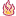## Brauche Hilfe bei Fallzahlberechung

Allgemeine Statistik mit R, die Test-Methode ist noch nicht bekannt, ich habe noch keinen Plan!

Moderatoren: EDi, jogo

Rneuling
Beiträge: 1
Registriert: So Feb 09, 2020 12:33 pm

###Brauche Hilfe bei Fallzahlberechung

Liebe R Gemeinde und Forumfreunde...

Folgende Aufgabe hätte ich zu erledigen:

Data are nicely normally distributed in each group, all have the same standard deviation and are independent from each other. Assume that Power analysis should be simulation based as described in Section 5.2.5 of the Lazic book. Consider a significance level of α = 0.05 and perform calculations both for power of 80%.

Assume you have four different treatments you would like to compare and you are interested in all pairwise differences of mean values. How many animals per group do you need to detect a pairwise difference of 1.5 standard deviations between two groups?

Bisher habe ich folgendes erledigt:

Code: Alles auswählen

``````n <- 500
sd=0.7
mean_a=0.5
mean_b=1
mean_c=1.5
mean_d=2

set.seed(1)
A  <- rnorm(n, mean_a, sd)
B  <- rnorm(n, mean_b, sd)
C  <- rnorm(n, mean_c, sd)
D  <- rnorm(n, mean_d, sd)

df=data.frame(A, B, C, D)
dfs=stack(df)
colnames(dfs) <- "Values"
colnames(dfs) <- "Treatment"

boxplot(df\$`A`,df\$`B`,df\$`C`,df\$`D`,
main="Group Comparison",ylab="Difference",xlab="Treatment Group",
names=c("Treatment 1","Treatment 2","Treatment 3","Treatment 4"),
col=c('gold', 'darkgreen','blue','red'))

summary(XXX <- aov(Values ~ Treatment, data = dfs))
tukey <- TukeyHSD(XXX)